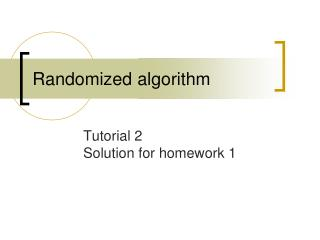Download PresentationRandomized algorithm

Loading in 2 Seconds...

# Randomized algorithm - PowerPoint PPT Presentation

Randomized algorithm. Tutorial 2 Solution for homework 1. Outline. Solutions for homework 1 Negative binomial random variable Paintball game Rope puzzle. Solutions for homework 1. Homework 1-1. step 1. ： a. ： c. Box 1. Box 2. ： b. ： d. Homework 1-1.I am the owner, or an agent authorized to act on behalf of the owner, of the copyrighted work described.
Download Presentation## Randomized algorithm

An Image/Link below is provided (as is) to download presentation

Download Policy: Content on the Website is provided to you AS IS for your information and personal use and may not be sold / licensed / shared on other websites without getting consent from its author.While downloading, if for some reason you are not able to download a presentation, the publisher may have deleted the file from their server.

- - - - - - - - - - - - - - - - - - - - - - - - - - E N D - - - - - - - - - - - - - - - - - - - - - - - - - -
Presentation Transcript
1. Randomized algorithm Tutorial 2Solution for homework 1

2. Outline • Solutions for homework 1 • Negative binomial random variable • Paintball game • Rope puzzle

3. Solutions for homework 1

4. Homework 1-1 step 1 ：a ：c Box 1 Box 2 ：b ：d

5. Homework 1-1 Draw a white ball from Box 2 : event A Pr(A) = Pr(A∩ (W∪B)) = Pr((A∩ W)∪(A∩ B))= Pr(A∩ W) + Pr(B∩ W) = Pr(A|W)Pr(W) + Pr(A|B)Pr(B)

6. Homework 1-1 • It is easy to see that

7. Homework 1-2 Wi = pick a white ball at the i-th time

8. Homework 1-2

9. Homework 1-2

10. Homework 1-2

11. Homework 1-2 1 b maps 1 a only For b=1, 1.36<a<2.36 means a=2 Pr(W1∩W2) = 2/3 * 1/2= 1/3 Then we may try b=2 →a=3 →odd b=4 →a=6 →even

12. Homework 1-3 By the hint X = sum is even. Y = the number of first die is even Z = the number of second die is odd Pr(X∩Y) = ¼ = ½ * ½ Pr(X∩Z) = ¼ = ½ * ½ Pr(Y∩Z) = ¼ = ½ * ½ Pr(X∩Y∩Z) =0

13. Homework 1-4 Let C1, C2, …, Ck be all possible min-cut sets.

14. Homework 1-5 i-th pair

15. Homework 1-5 Indicator variable Pr(Xi) = 1 if the i-th pair are couple. Pr(Xi) = 0 Otherwise Linearity of expectation X= Σi=1 to 20Xi E[X] = Σi=1 to 20E[Xi] = Σi=1 to 201/20 = 1

16. Homework 1-6

17. Homework 1-6

18. Homework 1-7 To choose the i-th candidate, we need i>m i-th candidate is the best [Event B] The best of the first i-1 candidates should be in 1 to m. [Event Y]

19. Homework 1-7 • Therefore, the probability that i is the best candidate is • The probability of selecting the best one is

20. Homework 1-7 Consider the curve f(x)=1/x. The area under the curve from x = j-1 to x = j is less than 1/(j-1), but the area from x = j-2 to x = j-1 is larger than 1/(j-1).

21. Homework 1-7

22. Homework 1-7 →g’(m)=0 when m=n/e →g’’(m)<0 , g(m) is max when m=n/e

23. Homework 1-7

24. Homework 1-8 (bonus) • S={±1, ±2, ±3, ±4, ±5, ±6} • X={1,-2,3,-4,5,-6} • Y={-1,2,-3,4,-5,6} • E[X]=-0.5 • E[Y]=0.5 • E[Z] = -3.5

25. Negative binomial random variable

26. Negative binomial random variable • Definition • y is said to be negative binomial random variable with parameter r and p if • For example, we want r heads when flipping a coin.

27. Negative binomial random variable • Let z=y-r

28. Negative binomial random variable • Let r=1 • Isn’t it familiar?

29. Negative binomial random variable • Suppose the rule of meichu game has been changed. The one who win three games first would be the champion. Let p be the probability for NTHU to win each game, 0<p<1.

30. Negative binomial random variable • Let the event • We know

31. Negative binomial random variable • where • Hence

32. Negative binomial random variable • Given that NTHU has already won the first game. What is the probability of NTHU being the champion?

33. Paintball game

34. Paintball game • You James Bond, and Robin Hood decide to play a paintball game. The rules are as follows • Everyone attack another in an order. • Anyone who get “hit” should quit. • The survival is the winner. • You can choose shoot into air.

35. Paintball game • James Bond is good at using gun, he can hit with probability 100%. • Robin Hood is a bowman, he can hit with probability 60%. • You are an ordinary person, can only hit with probability 30%. • The order of shot is you, Robin Hood then James Bonds.

36. Paintball game • What is your best strategy of first shoot? • Please calculate each player’s probability of winning.

37. Rope puzzle

38. Rope puzzle • We have three ropes with equal length (Obviously, there are 6 endpoint). • Now we randomly choose 2 endpoints and tied them together. Repeat it until every endpoint are tied.

39. Rope puzzle • There can be three cases • Which case has higher probability?